Image Analysis Quiz I, Non Verbal Reasoning

# Image Analysis Quiz I, Non Verbal Reasoning - SSC CGL

Test Description

## 20 Questions MCQ Test Non Verbal Reasoning - Image Analysis Quiz I, Non Verbal Reasoning

Image Analysis Quiz I, Non Verbal Reasoning for SSC CGL 2023 is part of Non Verbal Reasoning preparation. The Image Analysis Quiz I, Non Verbal Reasoning questions and answers have been prepared according to the SSC CGL exam syllabus.The Image Analysis Quiz I, Non Verbal Reasoning MCQs are made for SSC CGL 2023 Exam. Find important definitions, questions, notes, meanings, examples, exercises, MCQs and online tests for Image Analysis Quiz I, Non Verbal Reasoning below.
Solutions of Image Analysis Quiz I, Non Verbal Reasoning questions in English are available as part of our Non Verbal Reasoning for SSC CGL & Image Analysis Quiz I, Non Verbal Reasoning solutions in Hindi for Non Verbal Reasoning course. Download more important topics, notes, lectures and mock test series for SSC CGL Exam by signing up for free. Attempt Image Analysis Quiz I, Non Verbal Reasoning | 20 questions in 40 minutes | Mock test for SSC CGL preparation | Free important questions MCQ to study Non Verbal Reasoning for SSC CGL Exam | Download free PDF with solutions
 1 Crore+ students have signed up on EduRev. Have you?
Image Analysis Quiz I, Non Verbal Reasoning - Question 1

### Find out which of the figures (1), (2), (3) and (4) can be formed from the pieces given in figure (X).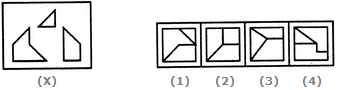Image Analysis Quiz I, Non Verbal Reasoning - Question 2

### Find out which of the figures (1), (2), (3) and (4) can be formed from the pieces given in figure (X).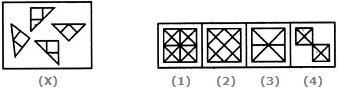Image Analysis Quiz I, Non Verbal Reasoning - Question 3

### Find out which of the figures (1), (2), (3) and (4) can be formed from the pieces given in figure (X).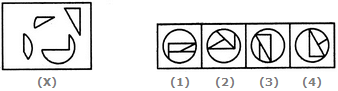Image Analysis Quiz I, Non Verbal Reasoning - Question 4

Find out which of the figures (1), (2), (3) and (4) can be formed from the pieces given in figure (X).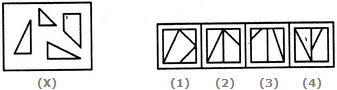Image Analysis Quiz I, Non Verbal Reasoning - Question 5

Find out which of the figures (1), (2), (3) and (4) can be formed from the pieces given in figure (X).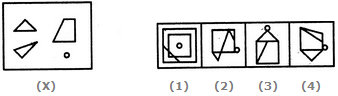Image Analysis Quiz I, Non Verbal Reasoning - Question 6

Find out which of the figures (1), (2), (3) and (4) can be formed from the pieces given in figure (X).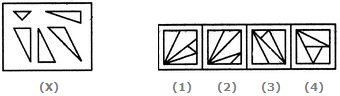Image Analysis Quiz I, Non Verbal Reasoning - Question 7

Find out which of the figures (1), (2), (3) and (4) can be formed from the pieces given in figure (X).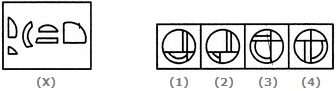Image Analysis Quiz I, Non Verbal Reasoning - Question 8

Find out which of the figures (1), (2), (3) and (4) can be formed from the pieces given in figure (X).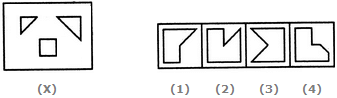Detailed Solution for Image Analysis Quiz I, Non Verbal Reasoning - Question 8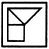Image Analysis Quiz I, Non Verbal Reasoning - Question 9

Find out which of the figures (1), (2), (3) and (4) can be formed from the pieces given in figure (X).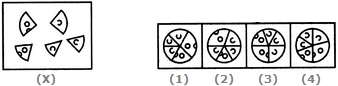Image Analysis Quiz I, Non Verbal Reasoning - Question 10

Find out which of the figures (1), (2), (3) and (4) can be formed from the pieces given in figure (X).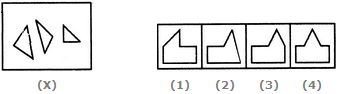Image Analysis Quiz I, Non Verbal Reasoning - Question 11

Find out which of the figures (1), (2), (3) and (4) can be formed from the pieces given in figure (X).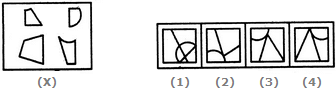Image Analysis Quiz I, Non Verbal Reasoning - Question 12

Find out which of the figures (1), (2), (3) and (4) can be formed from the pieces given in figure (X).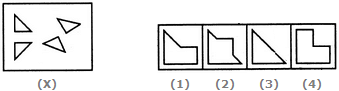Detailed Solution for Image Analysis Quiz I, Non Verbal Reasoning - Question 12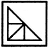Image Analysis Quiz I, Non Verbal Reasoning - Question 13

Find out which of the figures (1), (2), (3) and (4) can be formed from the pieces given in figure (X).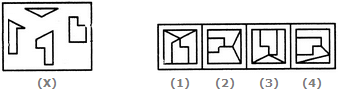Image Analysis Quiz I, Non Verbal Reasoning - Question 14

Select the alternative in which the specified components of the key figure (X) are found.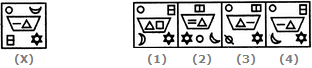Image Analysis Quiz I, Non Verbal Reasoning - Question 15

Select the alternative in which the specified components of the key figure (X) are found.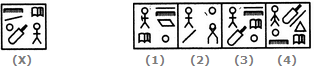Image Analysis Quiz I, Non Verbal Reasoning - Question 16

Select the alternative in which the specified components of the key figure (X) are found.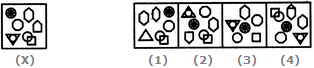Image Analysis Quiz I, Non Verbal Reasoning - Question 17

Select the alternative in which the specified components of the key figure (X) are found.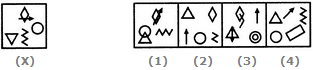Image Analysis Quiz I, Non Verbal Reasoning - Question 18

Select the alternative in which the specified components of the key figure (X) are found.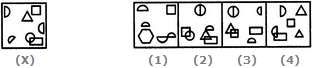Image Analysis Quiz I, Non Verbal Reasoning - Question 19

Select the alternative in which the specified components of the key figure (X) are found.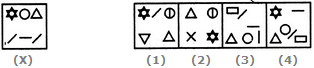Image Analysis Quiz I, Non Verbal Reasoning - Question 20

Select the alternative in which the specified components of the key figure (X) are found.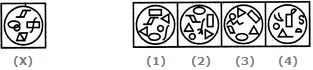## Non Verbal Reasoning

40 tests
Information about Image Analysis Quiz I, Non Verbal Reasoning Page
In this test you can find the Exam questions for Image Analysis Quiz I, Non Verbal Reasoning solved & explained in the simplest way possible. Besides giving Questions and answers for Image Analysis Quiz I, Non Verbal Reasoning, EduRev gives you an ample number of Online tests for practice

40 tests